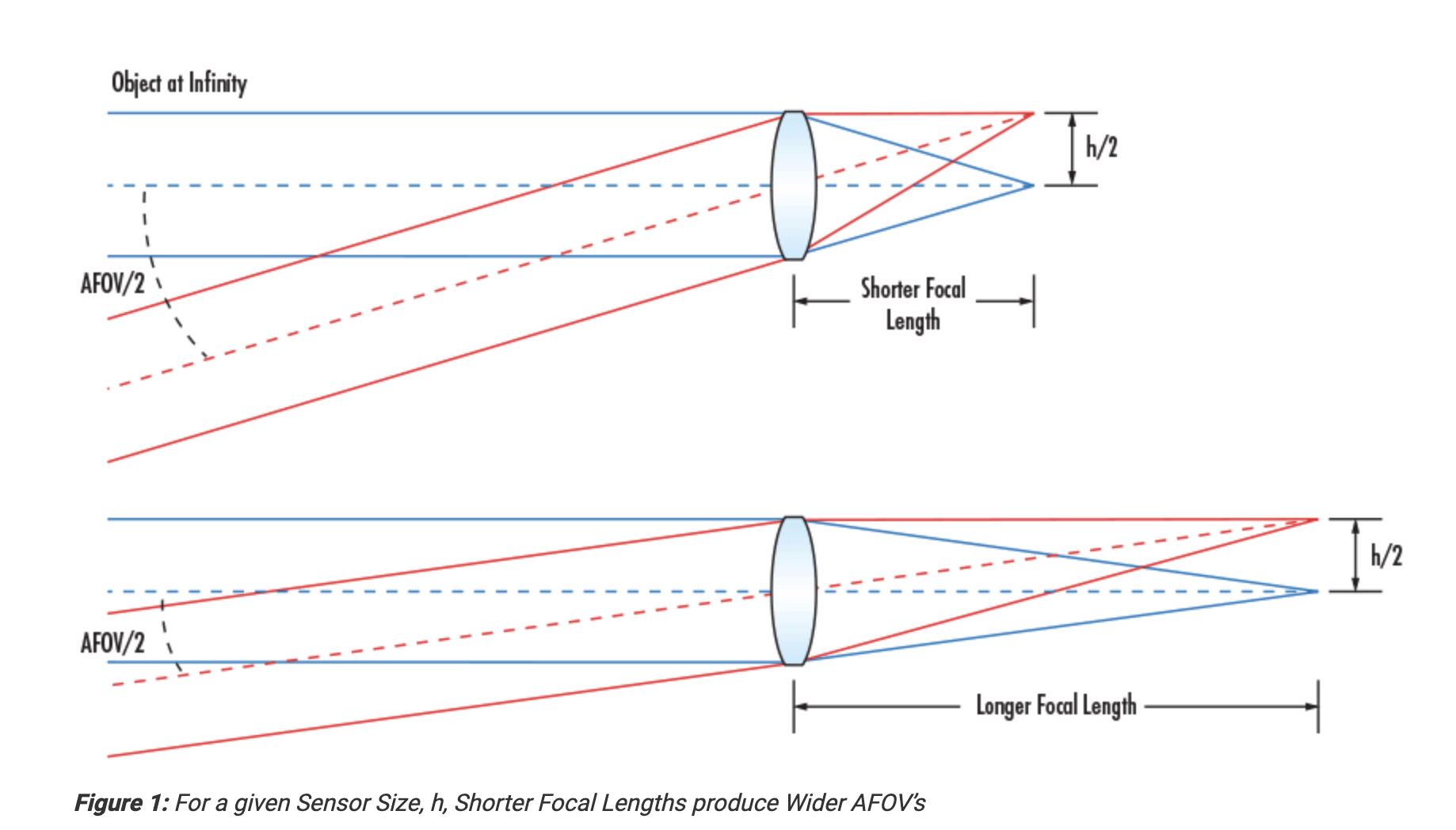# Augular Field of View(AFOV)

From here and here.

AFOV is typically specified as the full angle (in degrees) associated with the horizontal dimension (width) of the sensor that the lens is to be used with. Basically it means how wide(in degrees) a lens can see.Here is the equation how to calculate AFOV:

Here are some examples how to use the equation in Python:

Note that the “ / math.pi * 180” part is converting the result from in radians to in degrees.

Another interesting thing we can see from the 2 examples is that, “76mm lens + 60*60” has even a little bit bigger AFOV than “50mm lens + 36*24”, which though are very similar.